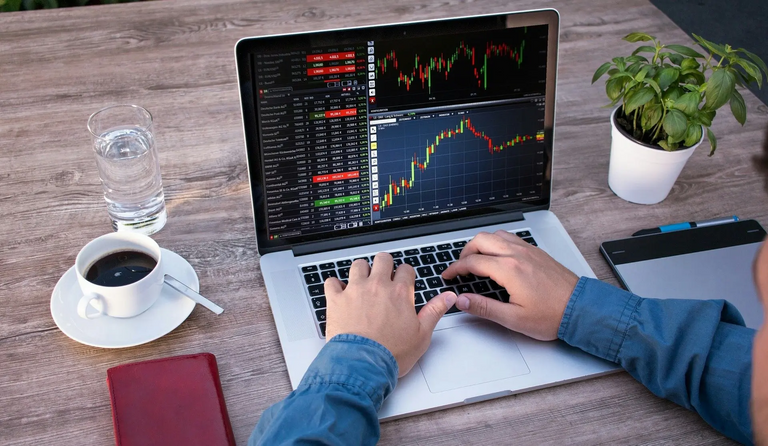# What are Pips?

in LeoFinancelast month

These are the points of interest of the price, which will allow you to calculate both your profits and your losses in specific distances traveled by the price. But to make it easier for us to learn it, we say that the Pips are the points of the market, these are very important because each operation is based on the pips, the gains or losses are measured with the pips.A note that should be taken into account is that the fifth decimal is not counted, that is a micro pips, this is one reason why many people make mistakes when calculating the pips, they start to be measured from the fourth decimal , that is to say.

1.20225 after the semicolon, the decimals come.
2 First decimal
0 Second decimal
2 Third decimal
2 Fourth decimal (pip)
5 Fifth decimal (micro pip that is not counted).

An example of a market movement is:

The price of the EUR / USD pair is at 1.20225 and then changed to 1.20235. It means that only one point moved. One pip of difference.

Another example is:

The AUD / USD pair is at 0.91451 and changed to 0.91551, change 10 points, that is, it moved 10 pips nothing more.
One last example.

If the price of the USD / CAD is at 1.25703 and it moves to 1.24705 it means that it moved 10 pips only.
With the Japanese Yen it is a bit different because the pip is the second decimal after the comma or period.
The EUR / JPY pair is at 126.828 and changed to 126.874, it means that it moved only 5 pips, it is the same as the previous ones but the decimal has been the second. In other words, the third is not counted.

If you did not understand something, you can ask me a question for the comments.

Posted Using LeoFinance Beta